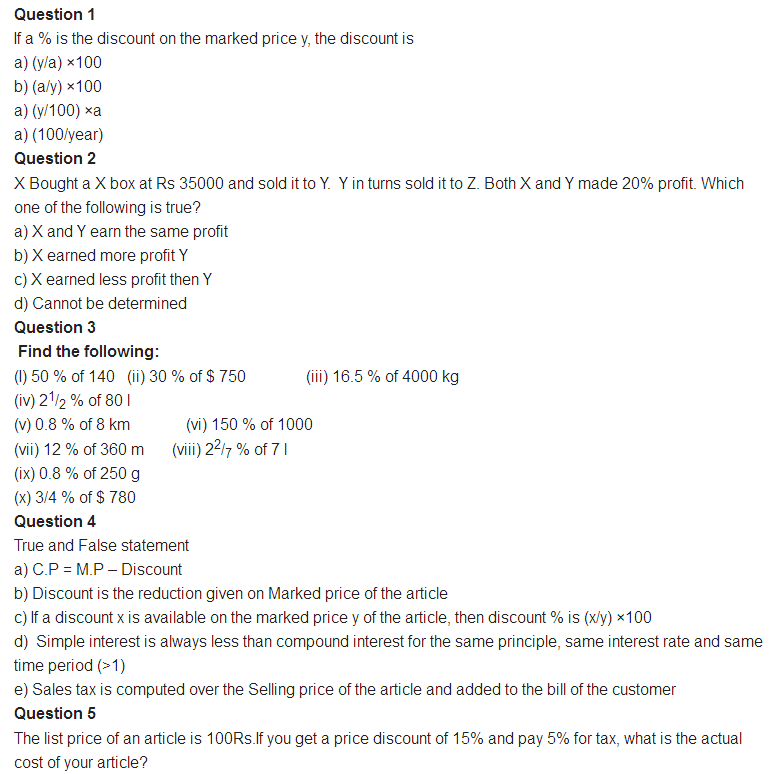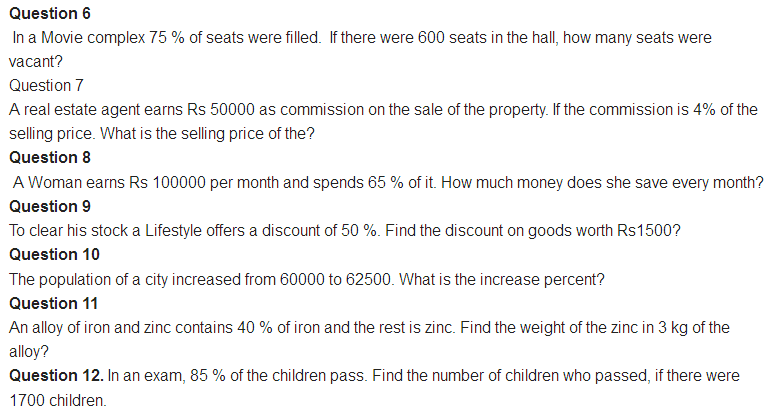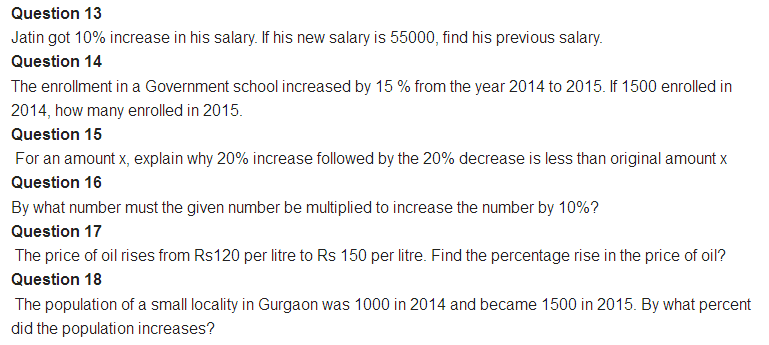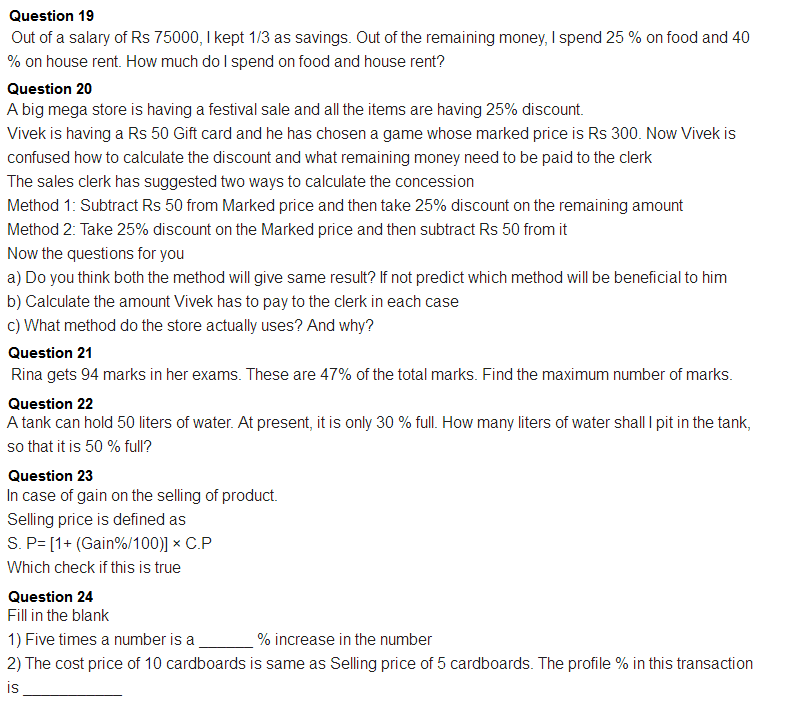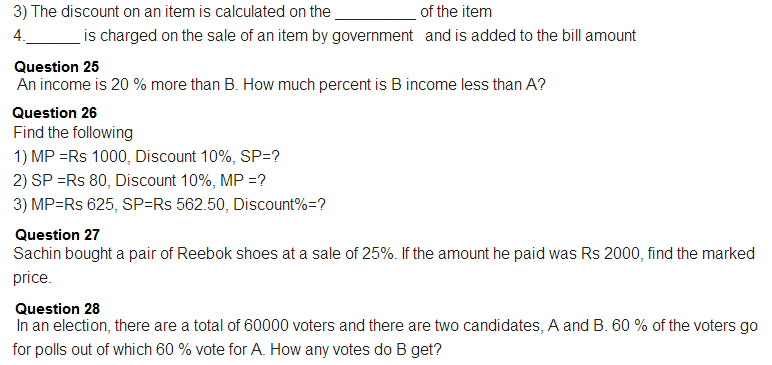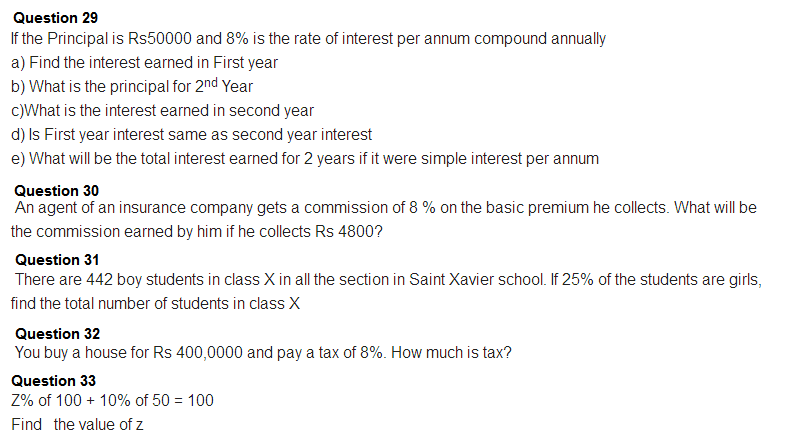# Important Question Class 8 Maths Chapter 8 Comparing Quantities

Important class 8 maths questions for chapter 8 comparing quantities provided here cover different variations of questions from this topic. These questions from comparing quantities will help the students to get completely acquainted with all the concepts from this chapter.

Also Check:

## Important Comparing Quantities Questions For Class 8 (Chapter 8)

Several types of questions from chapter 8 (NCERT) are provided here. There are both short answer type questions and long answer type questions including HOTS type questions to help students get thorough with all the topics and solve questions more effectively in the CBSE class 9 exam.

### Class 9 Chapter 8 Comparing Quantities Questions

1. Express 25% and 12% as decimals.

Solution:

25% = 25/100 = 0.25

12% = 12/100 = 0.12

2. Express 45% and 78% as a fraction.

Solution:

45% = 45/100 = 9/20

78% = 78/100 = 39/50

3. Calculate the ratio of 5 m to 20 km.

Solution:

First, make both the units the same.

So, 20 km has to be converted to “m”.

=> 20km = (20 × 1000)m

Thus, the ratio of 5 m to 20 km = 5/20000 = 1:4000

4. A student got 150 marks out of 200 in maths and got 120 marks out of 180 in science. In which subject did the student perform better?

Solution:

Express the marks in the form of ratios.

For maths, ratio = 150/200 = ¾

For science, ratio = 120/180= ⅔

Here, the ratio ¾ represents 75% (¾ × 100 = 75) and the ratio ⅔ represents 66.6% (⅔ × 100 = 66.6).

Thus, the student performed better in the maths exam.

5. If 72% of 25 students like maths, find out the number of students who do not like mathematics?

Solution:

It is given that 72% of 25 students like maths.

So, 72% of 25 = (72/100) × 25 = 18 students.

Now, from 25 students, 18 students like maths.

Thus, the number of students who do not like maths = 25 -18 = 7 students.

6. A person goes shopping and spends 75% of his money. If he is now left with Rs. 600, find out how much he had in the beginning.

Solution:

Let “x” be the initial amount that the had in the beginning.

From the question, the person spent 75% of x and is left with Rs. 600.

So, the amount he spent = x – 25

=> 75% of x = x – 600

=> (75/100) × x = x – 600

=> 75x = 100x – 60,000

=>25x = 60,000

Or, x = 2400.

Thus, the person had Rs. 2400 initially.

7. A shopkeeper bought two phones for Rs. 8,000 each. After selling the phones, there was a loss of 4% on the 1st phone while a profit of 8% on the 2nd phone. Calculate the overall gain or loss percent on the whole transaction.

Solution:

As the shopkeeper bought both phones at Rs. 8000 each, the total cost price = Rs. 16,000

Assume that the cost price of the 1st phone is Rs. 100

Consider the deal of phone 1,

As there is a 4% loss, selling price will be = Rs. 96

For CP = 100, SP = 96

So, for CP = 1, SP = 96/100

Now, when CP = 8000 (given), SP = 96/100 × 8000 = 7680

Thus, the selling price of 1st phone = Rs. 7680

Consider the deal of phone 2,

As there is an 8% profit, the selling price will be = Rs. 108

For CP = 100, SP = 108

So, for CP = 1, SP = 108/100

Now, when CP = 8000 (given), SP = 108/100 × 8000 = 8640

Thus, the selling price of 2nd phone = Rs. 8640

Here, the total selling price will be = Rs. 7680 + Rs. 8640 = 16320

Now, it can be seen that,

Total selling price > total cost price i.e. Rs. 16320 > Rs. 16000

So, there is a profit of Rs. (16320 – 16000) = Rs. 320

Now, the overall profit percentage will be-

Profit% = (Profit/Total Cost Price) × 100 = (320/16000) × 100 = 2

Thus, there is a total profit of 2% for the whole transaction.

8. In a certain school, there are 456 girls. Calculate the total number of students if 24% of the total students are boys.

Solution:

Assume the total number of students to be 100.

It is given that 24% are boys. So, total boys = 24% of 100 = 24

Thus, the total girls will be (100-24)% i.e. 76%.

So, the total girls = 76% of 100 = 76

But, it is given that there are 456 girls.

Now, for 76 girls, total students are 100

For, 1 girl, total students will be 100/76

And for 456 girls, total students will be 100/76 × 456 = 45600/76 = 600

Thus, the total number of students = 600.

### Extra Questions From Class 8 Chapter 8 Comparing Quantities